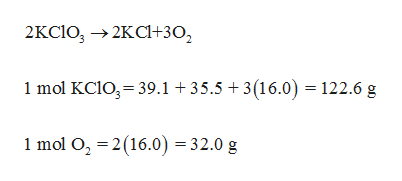# Small quantities of oxygen can be prepared in the laboratory by heating potassium chlorate, KClO3(s)KClO3(s) . The equation for the reaction is 2KClO3⟶2KCl+3O22KClO3⟶2KCl+3O2 Calculate how many grams of O2(g)O2(g) can be produced from heating 68.0 g KClO3(s)68.0 g KClO3(s) .STRATEGY:Convert the mass of KClO3KClO3 to moles.Convert the number of moles of KClO3KClO3 to the number of moles of O2O2 .Convert the number of moles of O2O2 to grams.Step 1: 68.0 g KClO368.0 g KClO3 is equal to 0.555 mol KClO30.555 mol KClO3 .Step 2: If 0.555 mol KClO30.555 mol KClO3 reacted, how much O2O2 was produced?

Question
6 views

Small quantities of oxygen can be prepared in the laboratory by heating potassium chlorate, KClO3(s)KClO3(s) . The equation for the reaction is

2KClO3⟶2KCl+3O22KClO3⟶2KCl+3O2

Calculate how many grams of O2(g)O2(g) can be produced from heating 68.0 g KClO3(s)68.0 g KClO3(s) .

STRATEGY:

1. Convert the mass of KClO3KClO3 to moles.

2. Convert the number of moles of KClO3KClO3 to the number of moles of O2O2 .

3. Convert the number of moles of O2O2 to grams.

Step 1: 68.0 g KClO368.0 g KClO3 is equal to 0.555 mol KClO30.555 mol KClO3 .

Step 2: If 0.555 mol KClO30.555 mol KClO3 reacted, how much O2O2 was produced?

check_circle

Step 1

The moles of KClO3 can be calculated as,

The...help_outlineImage Transcriptionclose2KCIO 2KC302 1 mol KC1O3 39.1 +35.5 3(16.0) 122.6 g 1 mol O2 2(16.0) =32.0 g fullscreen

### Want to see the full answer?

See Solution

#### Want to see this answer and more?

Solutions are written by subject experts who are available 24/7. Questions are typically answered within 1 hour.*

See Solution
*Response times may vary by subject and question.
Tagged in

### Inorganic Chemistry